# Jim's Technical Combustion Spud Gun: Temperature Study In A Closed Chamber

Continuing on with interesting things that can be learned with a closed combustion chamber burning propane and air, we will see if it is possible to measure the temperature of the combustion gases as the chamber is fired.

To measure the temperature we will examine the acoustics of the chamber. An air filled pipe, closed at both ends, resonates at a predictable frequency. This resonant frequency is a function of the length of the pipe, whether or not the ends are closed, and the temperature of the air in the pipe.

## Measurements

Our data set will be the piezo transducer signal measurement from the combustion of stoichiometric propane in air in a closed combustion chamber.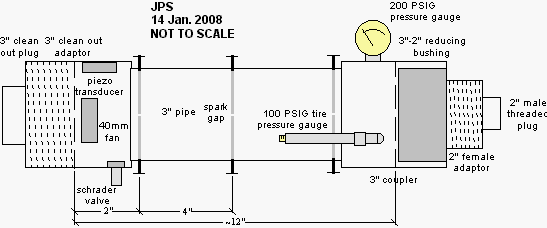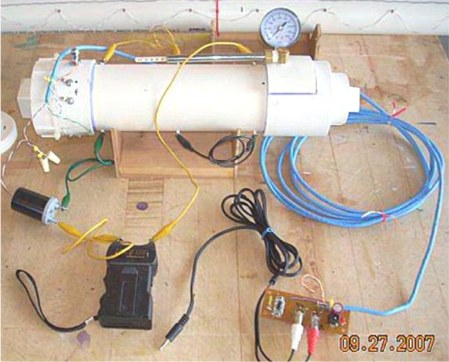The measurements were made with the chamber fan running and three spark gaps. For more details see my closed chamber page and the build log page.

## Calculations

### Resonant Frequency Of A Pipe

An air filled pipe closed at both ends resonates at a frequency given by;

f = nv / 2L    (Eq. 1)

Where;

f is the frequency
n is an integer (1 = fundamental, >1 for overtones)
v is the speed of sound
L is the length of the pipe.

The combustion chamber does not have nice flat ends. Both ends are capped with threaded plugs which give complex shapes at the ends of the pipe. Therefore, instead of attempting to measure the length of the chamber directly, I smacked the end of the chamber with a hammer and recorded the audio signal produced by the piezo transducer. Below is a screen shot of the Audacity window showing several smacks. The inlay is a spectrum analysis of the last smack (the highlighted region). Under these conditions the chamber resonated at a frequency of 440Hz.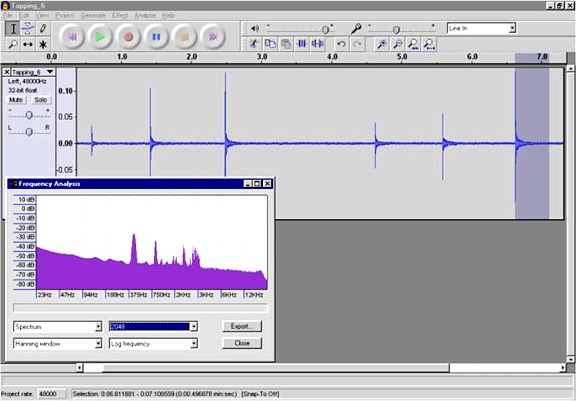Here is a wav file of the last tap.

I also pressurized the chamber to ~100 PSIG and recorded the tapping. (The speed of sound is independent of the pressure.) The pressurized chamber resonates at about the same frequency, 450 Hz this time, but with much better tonal quality.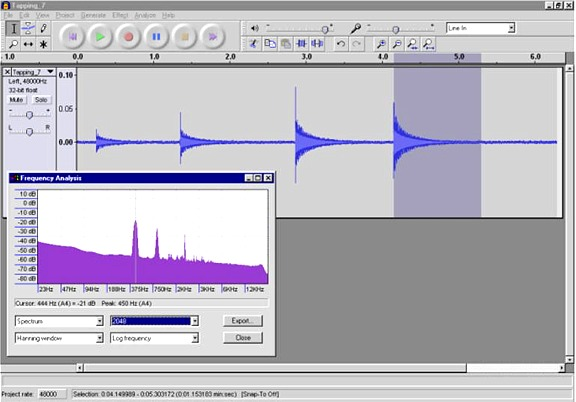Here is a wav file of the last tap. The sound recorded by the piezo transducer sounds pretty good, similar to a bell being rung. Note that the wav file is not what it sounds like to me as I smack the chamber. To me it just sounds like a hammer striking a hunk of PVC. Inside the chamber is the only place where the bell like sound can be heard since there are no openings in the chamber. For the same reason the chamber makes very little noise when fired, it makes very little noise on the outside when struck.

The temperature in my "lab" while doing this was 45F (7.2C). The speed of sound in air at this temperature was calculated with the commonly used equation;

vt = v0(sqrt(1+ Tc/273.15))     (Eq. 2)

Where;

Tc is the temperature in degrees celsius
v0 is the speed of sound in dry air at 0C, 331.4m/s
vt is the speed of sound at temperature Tc in m/s

Using Tc of 7.2C we get  vt = 335.7m/s (1101.5FPS).

Using the resonant frequency of the chamber and the speed of sound at this temperature, we can calculate an "acoustic length" for the chamber by solving equation 1 for L.

L = nv / 2f

Plugging in v=1101.5FPS, f=450Hz  and n=1 gives an "acoustic length" for the chamber of 1.224 feet (14.7 inch). The actual overall length of the chamber is 15 inches.

### Speed Of Sound As A Function Of Temperature In The Combustion Mix

The speed of sound in the combustion products for propane in air was calculated using GasEq. Starting with propane in air at a temperature of 10C (50F) the peak combustion temperature was calculated using GasEq's "Adiabatic T and composition at defined V" calculation. The change in the speed of sound (vsound) in the combustion products was then calculated using GasEq's "Equilibrium at defined T and constant V" calculation as the temperature was lowered in seven steps to 500K (227C). The vsound was plotted versus the celsius temperature (Tc) in Excel and fit with a fourth order polynomial. The resulting equation is;

Tc = (-6.497x10-8)vsound3 + (6.886x10-4)vsound2 - (0.9200)vsound + 3076,  R2 = 1.000  (Eq 3)

Where;

Tc is the gas temperature in degrees celsius
vsound is the speed of sound in FPS.

Equation 3 allows us to calculate the temperature of the gases from the speed of sound in the gases.

### Temperature in The Combustion Chamber

We are now ready to calculate the temperature of the combustion gases based on the recording of the piezo transducer's signal during firing of the chamber.

To estimate the temperature in the chamber at various times I took windows of the piezo recording ten cycles wide and measured the time. The average frequency within the window is f = 10/(window width in seconds). The time at the center of the window was used as the time for the particular measurement. From the frequency, and the acoustic length of the chamber, the speed of sound within the chamber can be calculated with equation 1 after solving for the speed of sound (v);

v = 2f L / n

Where the variables are as defined before. n is assumed to be 1, L is 1.224 feet (the chamber's acoustic length) and f is the frequency measured within the 10 cycle windows.

v = (2)(1.224ft)f
v = (2.448ft)f

From the speed of sound in the chamber the temperature of the gases can be calculated using the GasEq derived values using equation 3.

The graph below shows a region of the piezo transducer recording. The left side of the graph corresponds to the maximum piezo signal. The 10 cycle boxes are outlined in red. The values for each box are the observed frequency, the time to the center of the box, the calculated speed of sound (v) in FPS and the calculated temperature in degrees Celsius. In the vicinity of the peak piezo signal the signal is too complex to measure the frequency, the earliest frequency I could measure was at 32.8mS after ignition, about 8mS after the peak piezo signal.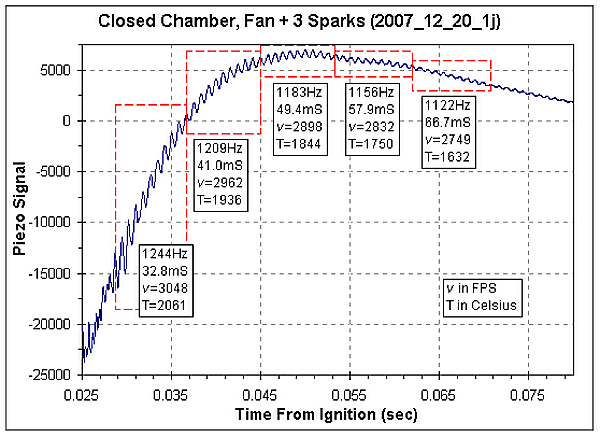According to GasEq, the peak adiabatic temperature for the combustion of propane in air with a starting temperature of 10C is 2358C. The maximum observed temperature, based on the chamber's acoustics, was 2061C. Since this value was measured 8mS after the peak piezo signal it probably is a bit lower than the actual peak temperature in the chamber.

The time versus temperature data from the graph above, along with a few additional time points, is summarized in the table below.

 Time From Ignition (mS) Time From Piezo Max (mS) Frequency (Hz) Speed of Sound (FPS) Temperature (C) 32.8 7.8 1244 3048 2061 41.0 16.0 1209 2962 1936 49.4 24.4 1183 2898 1844 57.9 32.9 1156 2832 1750 66.7 41.7 1122 2749 1632 78.0 53.0 1113 2727 1602 104.2 79.2 1056 2587 1412

Below is a graph of the calculated temperature versus time from ignition. Extrapolating the temperature to the piezo peak time (marked with the red line) indicates a temperature of 2200C at the time when the piezo signal was maximum.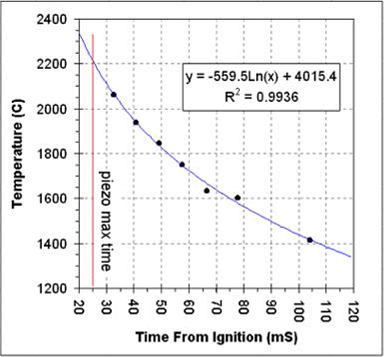The temperature appears to drop very quickly. For the seven measurements shown in the table the temperature appears to drop 649C in just 71 milliseconds. Based on the time and temperature drop we can estimate a thermal half-life of this chamber of about 110mS. So, for every 110mS after the temperature maximum the temperature drops by a factor of two.  For example, at 355mS after ignition (three t1/2's plus 25mS from ignition to the piezo's maximum signal), the temperature in the chamber will have dropped by about 88%.

We can use the Ideal Gas Law to relate the temperature in the chamber to the pressure in the chamber;

PV = nRT

Since the volume (V), number of moles of gas (n) and the gas law constant (R) are all constants for the closed chamber once combustion is complete, we can simplify the gas law to;

P = kT

Where k is a proportionality constant. Therefore the pressure in the chamber is proportional to the temperature in the chamber and the pressure drops at the same rate as the temperature. So, within a couple of tenths of seconds after combustion is complete the pressure in the chamber will have dropped back to near atmospheric pressure.

Since the energy  in the chamber is proportional to the temperature of the gases, the energy in the chamber drops at the same rate. Within a couple tenths of a second after ignition virtually all of the energy in the chamber has been lost as heat transferred to the chamber walls.

### Second Data Set

The calculations above were done using the combustion recording obtained with the chamber's fan running. To see if the fan makes a significant difference in the rate of heat loss I have applied the same analysis to a recording of the chamber fired with the fan off. The fuel was injected, the fan was run for a minute or so, the fan was then turned off and the chamber left to sit for 5 minutes (to allow the gases to stop moving) and then fired. For this firing only the central spark gap was used.

The table and graph below gives the acoustically calculated temperature versus time plot for this second set of data.

 Time From Ignition (mS) Time From Piezo Max (mS) Frequency (Hz) Speed of Sound (FPS) Temperature (C) 43.60 4.76 1203.4 3057 2074 51.75 12.92 1250.0 3175 2249 59.91 21.08 1203.4 3057 2074 68.29 29.46 1182.0 3002 1994 76.80 37.97 1168.2 2967 1943 85.46 46.63 1142.9 2903 1850 94.29 55.46 1121.1 2848 1771 103.37 64.54 1083.4 2752 1637 112.74 73.91 1051.5 2671 1525 130.50 91.67 1035.2 2629 1468 140.21 101.38 1025.6 2605 1436 150.06 111.23 1004.0 2550 1362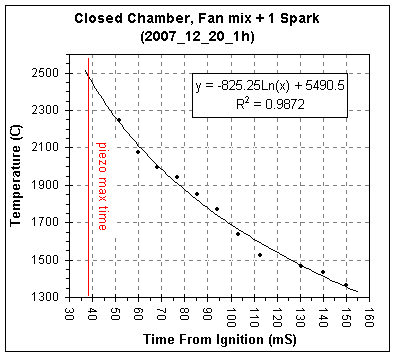The chamber burns slower without the fan running and with a single spark, the piezo's maximum signal occurring 39mSec after ignition versus 25mSec for combustion with the fan running and three sparks.

The estimated t1/2 for heat loss without the fan running is 114mS, essentially identical to the 110mS measured for the chamber with the fan running. (For more information on the closed chamber combustion studies see my Closed Chamber Studies age.)

### Video Of The Pressure Gauge During Firing

To confirm the above results, and to further examine the temperature and pressure drop I have used a digital still camera's video mode to record the movement of the pressure gauge's needle during firing.

For this chamber firing the tire pressure gauge was removed since that gauge tends to leak. A leak test of the chamber indicated that the leak rate was about 1 PSIG per minute at 100 PSIG. The chamber fan was not running for this firing, though it was used to mix the gases.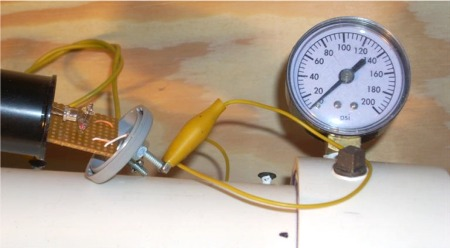The spark detector was pulled out of it's case (on the left side of the photo) in the hope that the video would record the ignition spark. Unfortunately, either the spark is to dim, or lasts for too short a time, to be recorded by the camera. The data recordings for this spark setup give a spark duration is only about 50 uSec, roughly 1/700th the video frame rate.

The gauge's response was recordable and the movie file is here. The image below shows 32 video frames recorded during the firing of the chamber. The camera's nominal frame rate is 30 FPS (one frame every 33.3mSec). In the first two frames the gauge's needle hasn't moved. In the third frame the needle is a blur in the range of 80 to 100 PSIG. In the next frame the needle is less blurry and the pressure has already dropped to about 76 PSIG. Over the next 24 frames (0.8 seconds) the needle is sharp in the images and drops down to 8 PSIG, which is the minimum reading of this gauge.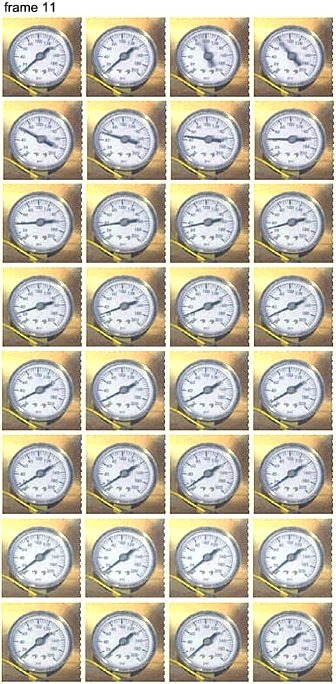The peak pressure in the chamber is difficult to determine from the video. The blurred needle in the third frame spans the range of 80 to 100 PSIG. It would seem that the upper end of this range, 100 PSIG, would be the maximum pressure during the exposure for that frame. Since the camera's shutter is not open for the entire time length of the frame it is likely that the actual peak pressure was missed. In addition, this particular gauge reads 10~15 PSIG low compared to the regulator gauge on my shop compressor. It seems likely then that the actual peak pressure in the chamber is perhaps 10 to 15 PSI greater than what is shown in the video.

Using the video, which is a bit sharper than the still images, I have estimated the gauge readings as a function of time. The times and pressures are shown in the table below.

 Frame # Time (Sec) Gauge Reading (PSIG) Frame # Time (Sec) Gauge Reading (PSIG) 1 0 8 17 0.533 20 2 0.033 8 18 0.567 19 3 0.067 94 19 0.600 17 4 0.100 76 20 0.633 16 5 0.133 62 21 0.667 15 6 0.167 52 22 0.700 14 7 0.200 46 23 0.733 13 8 0.233 41 24 0.767 12 9 0.267 36 25 0.800 11 10 0.300 34 26 0.833 10 11 0.333 32 27 0.867 9 12 0.367 28 28 0.900 8 13 0.400 27 29 0.933 8 14 0.433 25 30 0.967 8 15 0.467 23 31 1.000 8 16 0.500 21 32 1.033 8

The graph below shows a plot of this data. The red data points were not used in the curve fits.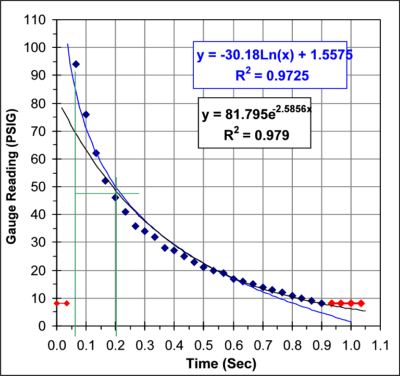From this data I get a pressure half-life of 129mSec. This agrees reasonably well with the 110-114 mSec half-life obtained from the acoustic studies.

### Systematic Errors in the Pressure Measurements

Looking at the graph of the pressure versus time you notice a systematic trend in the errors between the data points and the curve fit line. It appears that there may be two processes going on. The initial rate of heat loss appears to have a half-life of about 130 mSec. As the pressure drops the rate drops as well and towards the end the rate of heat loss has dropped to a half-life of about 300 mSec. It is possible that this trend is simply caused by the inability of the gauge, or the video, to respond quickly enough to the initial high rate of pressure change. Alternatively, this may represent a real characteristic of the heat loss in this system.

GasEq indicates that the thermal conductivity of the combustion gases at the adiabatic flame temperature is 0.15 W/m/K. Air at 25C has a thermal conductivity of about 0.023 W/m/K. As the gases in the chamber cool to room temperature the thermal conductivity drops by a factor of 6.5. It is possible that the systematic trend in the pressure versus time graph is caused by the increasing insulation properties of the combustion gases as they cool off. PVC has a thermal conductivity of about 0.19 W/m/K, similar to the thermal conductivity of the gases at the adiabatic flame temperature.

### Temperature Rise Of The PVC Chamber

Using the thermodynamic properties of PVC and the combustion gases we can estimate the net temperature rise of the PVC chamber.

The final temperature of the chamber and gases (TF) can be calculated from;

(Tg - TF)(Cg) = (TF - Tc)(Cc)

TF = (TcCc + TgCg) / (Cg + Cc)                        (Eq. 4)

The various values for equation 4 are given in the table below.

 Chamber Value Unit Variable Starting chamber temperature = 10 C Tc Mass of 12" of 3"ID PVC = 675 g Estimate of the mass of the chamber including fittings = 800 g Specific heat capacity PVC = 0.25 cal/g/C (mass PVC) x (specific heat capacity PVC) = 200 cal/C Cc Combustion Gases Starting temperature of gases (GasEq) = 2358 C Tg Volume of chamber = 1.74 L Density of the combustion products (GasEq) = 1.268 g/L Mass of combustion products = 2.21 g Specific heat capacity combustion products (Cv, GasEq) = 0.285 cal/g/C (mass gases) x (specific heat capacity gases) = 0.63 cal/C Cg Chamber to Gases Heat Capacity Ratio (mass PVC) x (specific heat capacity PVC) -------------------------------------------------------------------- (mass gases) x (specific heat capacity gases) = 318

So, for every 318°C drop in the gases' temperature the PVC chamber's temperature rises by just 1°C.

Using the values in the table above and equation 4 we can calculate the final temperature of the PVC and gases.

 Final Temperature Final temperature of gases and PVC = 17.4 C TF Temperature rise of the PVC = 7.4 C

So, even though the combustion gases' peak temperature is more than 2300C (~4200F), the final temperature of the gases and the PVC is only about 7.4C (13F) above the starting temperature.